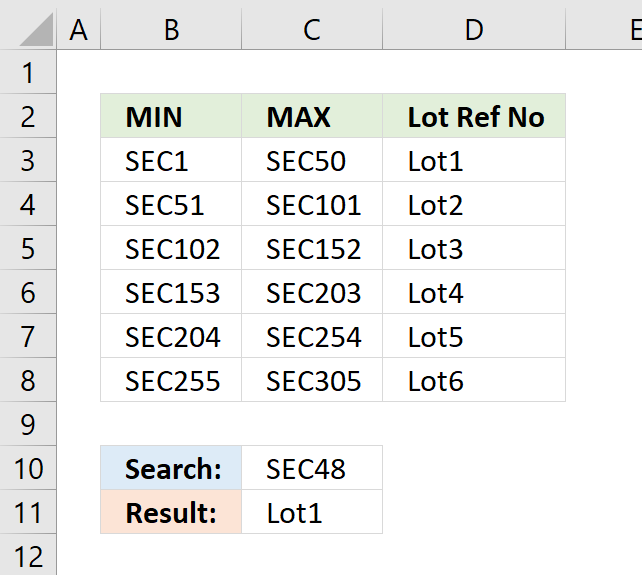Author: Oscar Cronquist Article last updated on November 18, 2021This article demonstrates how to match a value containing both text and digits to ranges. The search value is specified in cell C10, cell range B3:B8 contains the start values for the ranges and C3:C8 contains the end values for the ranges.

The formula matches the numerical part of the value in cell C10 to the start and end numbers for each range, it returns a corresponding value from cell range D3:D8.

For example, cell C10 contains SEC48. The numerical part is 48, 48 is larger than the numerical part in cell B3 and lower than C3. The corresponding value from D3:D8 is cell D3 which is returned to cell C11.

Array formula in cell C11:

=LOOKUP(MID(C10, 4, 999)*1, MID(B3:B8, 4, 999)*1, D3:D8)

### How to enter an array formula

This formula is an array formula. To enter an array formula, type the formula in a cell then press and hold CTRL + SHIFT simultaneously, now press Enter once. Release all keys.

The formula bar now shows the formula with a beginning and ending curly bracket telling you that you entered the formula successfully. Don't enter the curly brackets yourself.

### Explaining formula in cell C11

#### Step 1 - Extract number from search value

The MID function returns a given number of characters from a value. This allows us to extract only the numbers from the value.

MID(C10,4,999)

becomes

MID("SEC48",4,999)

and returns "48".

This is still a text value so in order to use that, we must first convert it to a numerical value.

MID(C10,4,999)*1

becomes

"48"*1

and returns 48.

#### Step 2 - Extract numbers from lookup range

The second argument in the LOOKUP function is the lookup list. This list must also be converted into numbers. I will use the same technique described in the previous step to extract and convert the numbers from cell range B3:B8.

MID(B3:B8,4,999)*1

becomes

MID({"SEC1"; "SEC51"; "SEC102"; "SEC153"; "SEC204"; "SEC255"}, 4, 999)*1

becomes

{"1";"51";"102";"153";"204";"255"}*1

and returns

{1;51;102;153;204;255}.

#### Step 3 - Return corresponding value on the same row as the matching value

The LOOKUP function matches the lookup value to a list of range values and returns the corresponding value. Remember that the list of values must be sorted in an ascending order for it to work.

LOOKUP(MID(C10,4,999)*1,MID(B3:B8,4,999)*1,D3:D8)

becomes

LOOKUP(48, {1; 51; 102; 153; 204; 255}, {"Lot1"; "Lot2"; "Lot3"; "Lot4"; "Lot5"; "Lot6"})

and returns "Lot1" in cell C11.

### Get Excel *.xlsx file

Match text and numbers combined.xlsx

### Alternative formula

=INDEX(\$D\$3:\$D\$8, MATCH(MID(C10, 4, 999)*1, MID(B3:B8, 4, 999)*1))